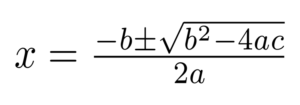Tuesday / October 19

# Crafting Quality Math Generalizations: Establishing Goals to Focus LearningOne of the Mathematics Teaching Practices from National Council of Teachers of Mathematics (NCTM, 2014) is:

Establish mathematics goals to focus learning. Effective teaching of mathematics establishes clear goals for the mathematics that students are learning, situates goals within learning progressions, and uses goals to guide instructional decisions.

Generalizations are summaries of thought and overriding ideas that we want our students to understand from their study. They are our mathematics goals to focus learning. Lynn Erickson (2007) first coined the term “generalizations” and in education circles generalizations are also known as enduring or essential understandings. It is a statement that connects two or more concepts in a sentence of relationship. Here are some examples of generalizations:

Students understand that:

• Linear functions show relationships that exhibit a constant rate of change
• A function represents a mapping (rule) that assigns each input (domain) with one output (range)
• For right-angled triangles, the area of the square drawn from the hypotenuse represents the sum of the areas of the squares drawn from the other sides
• Logarithm laws provide a means of changing multiplicative processes into additive processes and can be used to find inverses of exponential functions

If my students said any of the above statements I would be satisfied that they have fully understood the topic and actually have a deep understanding of the concepts involved.

To help craft quality generalizations Erickson (2007)  suggests a scaffolding process.

Here is a specific example of how to scaffold a generalization in order to  improve the quality of the generalization.

 Level 1 Students will understand that…..Logarithm laws affect logarithmic expressions Level 2 How or why? Logarithm laws reduce logarithmic expressions utilizing  the inverse process of exponential functions which represent continuous compounded growth. Level 3 So what? What is the significance or effect? Logarithm laws provide a means of changing multiplicative processes into additive processes and this can provide the means to find inverses of exponential functions (continuous compounded growth).

In summary here are the three levels for crafting generalizations:

 Level 1 Students will understand that… Level 2 How or why? (Choose which question is most appropriate) Level 3 So what? What is the significance or effect?

It is important to note here that critical understanding in mathematics is usually achieved through a level 2 generalization therefore the target for curriculum development and instruction is level 2 type of generalizations. Level 3 generalizations are used if we wish to extend an idea or apply to a real life situation.

How do we draw out conceptual understandings from our students?

John Mason (1996) encapsulates the essence of math teaching in the following quote: a lesson without the opportunity for learners to generalise is not a mathematics lesson”.

In a concept-based model of curriculum and instruction we do not tell students the generalizations at the beginning of a unit of work or at the beginning of a lesson. We guide students to communicate generalizations through a variety of strategies.

The following table illustrates some examples of how we can draw conceptual understandings from our students.

 Strategy Example Give the concepts and ask students to connect these concepts in a statement that reflects the purpose of the unit of study. Write the following concepts in a related sentence. This statement should demonstrate your understanding of how these concepts are connected: zeros of a function, roots of an equation, x intercepts, quadratic formula or parallel lines, gradient, transversal, angles At the end of a lesson or unit of work ask students to complete the sentence, “I understand that……..” I understand that solving a quadratic equation by using the quadratic formula graphically displays the x intercepts of a quadratic function. Give students a choice of a few statements and ask students to choose one that explains the big idea of the lesson or unit and to justify why. The quadratic formula isSolving a quadratic equation by using the quadratic formula graphically displays the x intercepts of a quadratic function. The quadratic formula tells us what x is equal to and allows us to solve quadratic equations. After a lesson or unit of study ask students to create a concept map, which includes the concepts they have learned and how they are connected with statements explaining this. With the word quadratics at the centre, create a concept map using the ideas you have learned and include statements to explain how they are connected. Give students a close activity where they have to fill in the blanks, and provide them with a list of hints. Hint Jar: quadratic equation quadratic formula quadratic Function x intercepts Solving a ___________  ________ by using the _________ ________ graphically displays the __________ of a ______________. Ask students to devise a headline which summarizes the key ideas (concepts) of a unit. This can be used as a diagnostic or formative assessment.

Wathall, J. T. H. (2016) Concept-based mathematics: Teaching for deep understanding in secondary classrooms. Thousand Oaks, CA: Corwin.

Crafting generalizations is an important part of unit planning and if teachers know what their end goals are for their students then the rest of the unit planning process is enhanced.

#### Tags

Jennifer Chang-Wathall is an independent consultant, author, faculty chair of mathematics at Island School and honorary faculty adviser and part time lecturer for the University of Hong Kong.

With over 20 years experience in the education field, Jennifer has worked in several international schools in various management roles. She is an international keynote speaker and presenter, delivering workshops on Concept-Based Mathematics, 21st Century Learning Skills, and Concept-Based Curriculum.

Jennifer works as an independent consultant helping math departments and schools transition to concept-based curriculum and instruction. She utilizes her skills as a certified Performance Coach to facilitate transition and change.

Her book Concept-Based Mathematics: Teaching for Deep Understanding in Secondary Classrooms was released in February 2016.